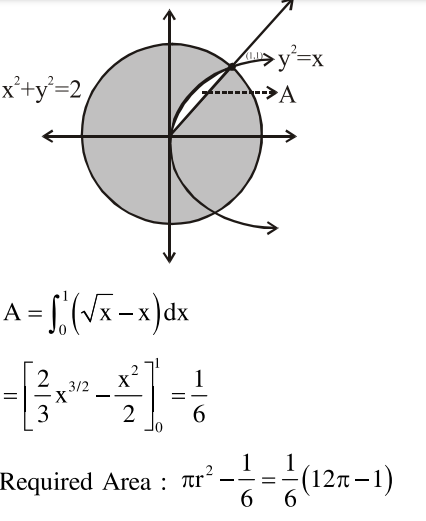# The area of the region,`
Question:

The area of the region, enclosed by the circle $x^{2}+y^{2}=2$ which is not common to the region bounded by the parabola $\mathrm{y}^{2}=\mathrm{x}$ and the straight line $\mathrm{y}=\mathrm{x}$, is :

1. $\frac{1}{3}(12 \pi-1)$

2. $\frac{1}{6}(12 \pi-1)$

3. $\frac{1}{6}(24 \pi-1)$

4. $\frac{1}{3}(6 \pi-1)$

Correct Option: , 2

Solution: